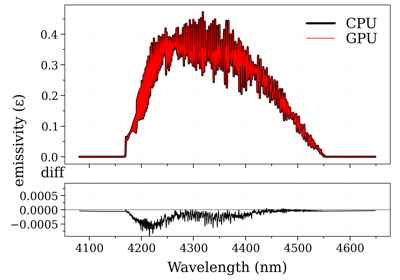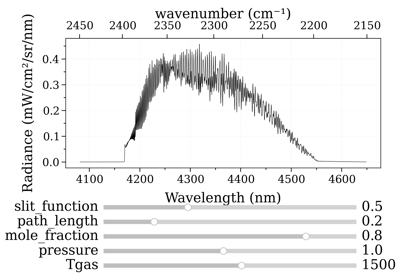# RADIS-GPU Spectrum Calculation¶

RADIS provides GPU acceleration to massively speedup spectral computations. The GPU code is written in CUDA-C++, which is compiled at runtime through the python package cupy. Because the CUDA code is compiled at runtime, a CUDA compiler such as NVRTC or NVCC must be installed before running the code. The easiest way to make sure a CUDA compiler is installed is by installing the Nvidia GPU computing toolkit, see: https://developer.nvidia.com/cuda-downloads. Currently only Nvidia GPU’s are supported by RADIS, and this is unlikely to change in the foreseeable future.

The GPU code requires some initialization functions that are run on the CPU (

The GPU computations are so fast that in almost all cases the total computation time is limited by the CPU-to-GPU (also called host-to-device) data transfer. As a result, computing consecutive spectra is extremely fast.

RADIS implements two functions that expose GPU functionality:

• `eq_spectrum_gpu()` computes a single spectrum and returns.

• `eq_spectrum_gpu_interactive()` computes a single spectrum and allows to interactively adjust parameters such as temperature, pressure, and composition, updating the spectrum in real time. Because the database only has to be transferred once, the updated spectra are calculated extremely fast.

By default both functions will be compiled an ran on a GPU if available. The CUDA code is written “architecture-agnosticly” which means it can be compiled for either GPU or CPU. It is therefore possible to use the same GPU functions without an actual GPU by passing the keyword `emulate=True`, which forces use of the CPU targeted compiled code. This feature is mostly for developers to check for errors in the CUDA code, but it can be used for interactive plotting on the CPU for small spectra.

GPU computation is currently only supported for equilibrium spectra. It is likely that non-equilibrium spectra will be supported at some point in the future.

## Single Spectrum¶

As mentioned above, the function `eq_spectrum_gpu()` produces a single equilibrium spectrum using GPU acceleration. Below is a usage example:

```sf = SpectrumFactory(
2100,
2400,  # cm-1
molecule="CO2",
isotope="1,2,3",
wstep=0.002,
)

sf.fetch_databank("hitemp")

s = sf.eq_spectrum_gpu(
Tgas=1100.0,  # K
pressure=1,  # bar
mole_fraction=0.8,
path_length=0.2,  # cm
emulate=False,  # runs on GPU
)

s.apply_slit(0.5)#cm-1
```

### Examples using `radis.lbl.SpectrumFactory.eq_spectrum_gpu`¶GPU Accelerated Spectra

GPU Accelerated SpectraReal-time GPU Accelerated Spectra (Interactive)

Real-time GPU Accelerated Spectra (Interactive)

## Interactive Spectrum¶

Computing the first GPU spectrum in a session takes a comparatively long time because the entire line database must be transferred to the GPU. The real power of GPU acceleration becomes evident when computation times are not limited by data-transfer, i.e., when multiple consecutive spectra are synthesized. One obvious use case would be the fitting of a spectrum. Another one is interactive plotting, which can be done by calling `eq_spectrum_gpu_interactive()`. A usage example is shown below:

```from radis.tools.plot_tools import ParamRange
sf = SpectrumFactory(
2100,
2400,  # cm-1
molecule="CO2",
isotope="1,2,3",
wstep=0.002,
)

sf.fetch_databank("hitemp")

s = sf.eq_spectrum_gpu_interactive(
Tgas=ParamRange(300.0, 2500.0, 1100.0),  # K
pressure=ParamRange(0.1, 2, 1),  # bar
mole_fraction=ParamRange(0, 1, 0.8),
path_length=ParamRange(0, 1, 0.2),  # cm
slit_FWHM=ParamRange(0, 1.5, 0.24),  # cm-1
emulate=False,  # runs on GPU
plotkwargs={"nfig": "same", "wunit": "nm"},
)
```

Note that `eq_spectrum_gpu_interactive()` takes the place of all `eq_spectrum_gpu()`, `s.apply_slit()`, and `s.plot()` seen in the earlier example, and for this reason the syntax is a little bit different. For example, we directly pass the `var` keyword to `eq_spectrum_gpu_interactive()` to specify which spectrum should be plotted, and keyword arguments to `s.plot()` are passed through `plotkwargs`.

Quantities that are to be varied must be initialized by a `ParamRange()` (valmin, valmax, valinit) object, which takes the minimum value, maximum value, and init values of the scan range. Each `ParamRange()` object will spawn a slider widget in the plot window with which the parameter can be

interactively adjusted. The algorithm is extremely fast for a large number of lines (>100M) and will update with very low latency (<200ms typically). The code is not currently optimized for large wavenumber ranges (>500cm-1) however, which may take a bit longer (up to a couple seconds), provided the GPU didn’t run out of memory.

At this moment the application of the instrumental function is done on the GPU and is limited to a Gaussian function. This will almost certainly be updated in the future to include other popular instrumental functions, including custom ones.